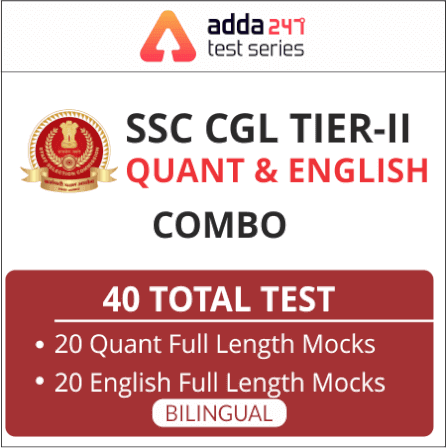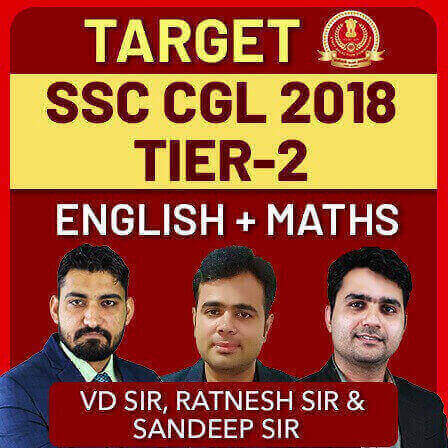# SSC CGL Mains Quantitative Aptitude Questions : 15th AugustDear aspirants,

As you all know, the upcoming months are lined up with various important exams like SSC CGL Mains 2018, so we are here to help you with the subject that is common to all of the given exams. We are providing daily quantitative aptitude quizzes, practice which will help you to score good marks in this section. We aim to provide the best study material to our readers with exam level questions to help them get used to the recent pattern. Attempt this quiz and check your preparation.

Q1. The average of 10 numbers is 40.2. Later it is found that two numbers have been wrongly added. The first is 18 greater than the actual number and second number added is 13 by mistake instead of 31. Find the correct average.
(a) 49.8
(b) 40.2
(c) 45.6
(d) 38.4
Ans.(b)
Sol. Average of 10 numbers = 40.2
∴ sum of 10 numbers = 40.2 × 10 = 402
As per Questions,
Actual Average = (402-18+(31-13))/10
= (402-18+18)/10
= 40.2

Q2. A bank pays 16.5% per annum as interest on its deposits while it charges 20% per annum as interest from its borrowers. If at the end of the years the bank earns Rs. 10.5 crore. Find how much money was transacted during the year?
(a) 300 crore
(b) 320 crore
(c) 412 crore
(d) None of these
Ans.(a)
Sol. Let the bank makes a transaction of Rs. x crores.
According to question:-
(20 – 16.5)% of x = 10.5 crore
3.5/100×x=10.5
∴x=(10.5×100)/3.5=300 crore

Q3. At his usual rowing rate, Ram can travel 12 miles downstream in a certain river taking 6 hour less than that taken by him to travel the same distance upstream. But if he could double his usual rowing rate for his 24 mile round trip, the downstream 12 miles would then take only one hour less than the upstream 12 miles. What is the speed of the current in miles per hour?
(a) 1.5 mph
(b) 2 1/3 mph
(c) 1 1/3 mph
(d) 2 2/3 mph
Ans.(d)
Sol. Let Ram’s rowing rate is ‘x’
Speed of current is ‘y’
Downstream time taken = 12/(x+y)
Upstream time taken = 12/(x-y)
According to the question
12/(x-y)-12/(x+y)=6⇒x^2-y^2=4y __________(i)
Now, If speed of boat doubles ⇒ ‘2x’
Time is 1hr. less as compared to upstream
12/(2x-y)-12/(2x+y)=1⇒4x^2-y^2=24y __________(ii)
From (i) & (ii) we get
y = 8/3 mph.

Q4. A man borrows Rs. 1000 and agrees to repay it with a total interest of Rs. 140 in 12 installments, each installment being less than the one preceding it by Rs. 10. What should be his first installment?
(a) 160
(b) 80
(c) 150
(d) 40
Ans.(c)
Sol. The total amount = Rs. [1000+140]
= Rs. 1140
Let the 1st installment = Rs. x According to question,
1140=12/2 [2x+(12-1)(-10)]
1140/6=[2x-110]
190 = 2x – 110
x = (190+110)/2=150
Ist installment = Rs. 150

Q5. A train’s journey is disrupted due to an accident on its track after it has travelled 30 km. Its speed then comes down to 4/5 th of its original and consequently it runs 45 min late. Had the accident taken place 18 km farther away, it would have been 36 min late. Find the original speed of the train.
(a) 25 km/hr
(b) 36 km/hr
(c) 30 km/hr
(d) 20 km/hr
Ans.(c)
Sol.
Case I: When accident occurs at 30 kms.
Usual time taken by train to cover remaining distance =45×4=180 min = 3 hr.
Case II : When accident occurs at 48 kms.
Usual time taken by train to cover remaining distance = 36 × 4 = 144 min
In (180 – 144) = 36 min., train covers 18 kms.
Hence speed of train = 18/36×60=30 km/hr

Q6. Population of a district is 2,96,000 of which 1,66,000 are males. 50% of the population is literate. If 70% males are literate, then the number of women who are literate is?
(a) 32,900
(b) 31,800
(c) 35,295
(d) 30,945
Ans.(b)
Sol. Population of literates = 50% of 296000
= 0.50 × 296000
= 148000
No. of males literates = 70% of 166000
= 0.7 × 166000
= 116200
No. of female literates = 148000 – 116200
= 31800

Q7. Weights of two friend Ram and Shyam are in the ratio of 4 : 5. Ram’s weight increases by 10% and the total weight of Ram and Shyam together becomes 82.8 kg., an increase of 15%. By what percent did the weight of Shyam increase?
(a) 19
(b) 20
(c) 15
(d) 17
Ans.(a)
Sol. Let Ram and Shyam weights are
= 4x and 5x respectively
Now:-
Their previous weight (sum):-
82.8/115×100=72 kg
According to question:-
⇒5x+4x=72
∴ x = 8
∴ Ram’s weight = 8 × 4 = 32 kg
Shyam’s weight = 5×4=40 kg
Their increased weight
= [10.8-3.2]
= 7.6 kg
∴ % increase = 7.6/40×100=19%

Q8. Mr. Maitray invested money in two schemes A and B offering C.I. at the rate of 8 p.c.p.a. & 9 p.c.p.a. respectively. If the total amount of interest incurred through the two schemes together in two years was Rs. 4818.30 and the total amount invested was Rs. 27,000. What was the amount invested in scheme A?
(a) 12000
(b) 15000
(c) 24000
(d) 20000
Ans.(a)
Sol. Let amount invested in scheme A = Rs. x
Then in B = Rs. (27000 – x)
From allegation method,
Complete amount after 2 years at 8% = 4492.8
Complete amount after 2 years at 9% = 5078.7
But given that , amount after 2 years = 4818.3
So, interest = (5078.7 – 4818.3)= 260.4
and another interest amount=4818.3- 4492.8= 325.5
so, the ratio = 4/5
Amount of A = 4*27000/9 =12000

Q9. Train ‘A’ leaves Mumbai Central for Lucknow at 11 am running at the speed of 60km/h. Train ‘B’ leaves Mumbai Central for Lucknow by the same route at 2 pm on the same day, running at the speed of 72 km/h. At what time will the two trains meet each other?
(a) 11 : 30 am Sunday
(b) 11 pm Sunday
(c) 2 am Tuesday
(d) 5 am on Next day

Ans.(d)
Sol.Given that:-
Speed of A = 60 km/hr
Distance travelled in 3 hr = 60 × 3
= 180 km
At 2 : 00 pm:-
Speed of B = 72 km/hr
Time difference = 3 hrs
Relative velocity = [72-60]=12 km/hr
Now:- Time – gap (meeting)
= 180/12=15 hr after they meet
⇒ They will meet at 2 pm + 15 hour = 5 am

Q10. Two places A and B are 100 km apart on a highway. One car starts from A and another from B at the same time. If the cars travel in the same direction at a constant speed they will meet in 5 hours. If they travel towards each other, they will meet in 1 hr. What is the speed of the car running faster?
(a) 60 km/h
(b) 50 km/h
(c) 40 km/h
(d) 32 km/h

Ans.(a)
Sol.Let the speed of A = u km/hr
Speed of B = v km/hr
As per question:-
100/((u+v))=1 hr
(v+u) = 100 ………….(i)
Again from question:-
100/((u-v))=5,
5u-5v=100 ………….(ii)
From equation (i) and (ii):-
10u=600
u=60 km/hr

#### Check SSC CGL Tier 1 Expected Cut-off

You May Also Like To Read :